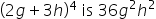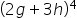Maths-
General
Easy

Question

# Dakota said the third term of the expansions of.Explain Dakota’s error and then correct the answer.Hint:

## The correct answer is: 4C2

### Step 1 of 2:The given expression is, here x = 2g & y = 3h.Thus, the expansion would be:Step 2 of 2:Analyzing the expansion, find the coefficient of  g2h2. The value is: 216 Dakota got the wrong answer because she did not count the value 4C2 .

You can use Both the Pascal’s triangle and binomial expansion to find the value of (x + y)n .#### With Turito Foundation.#### Get an Expert Advice From Turito.